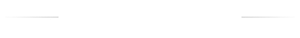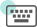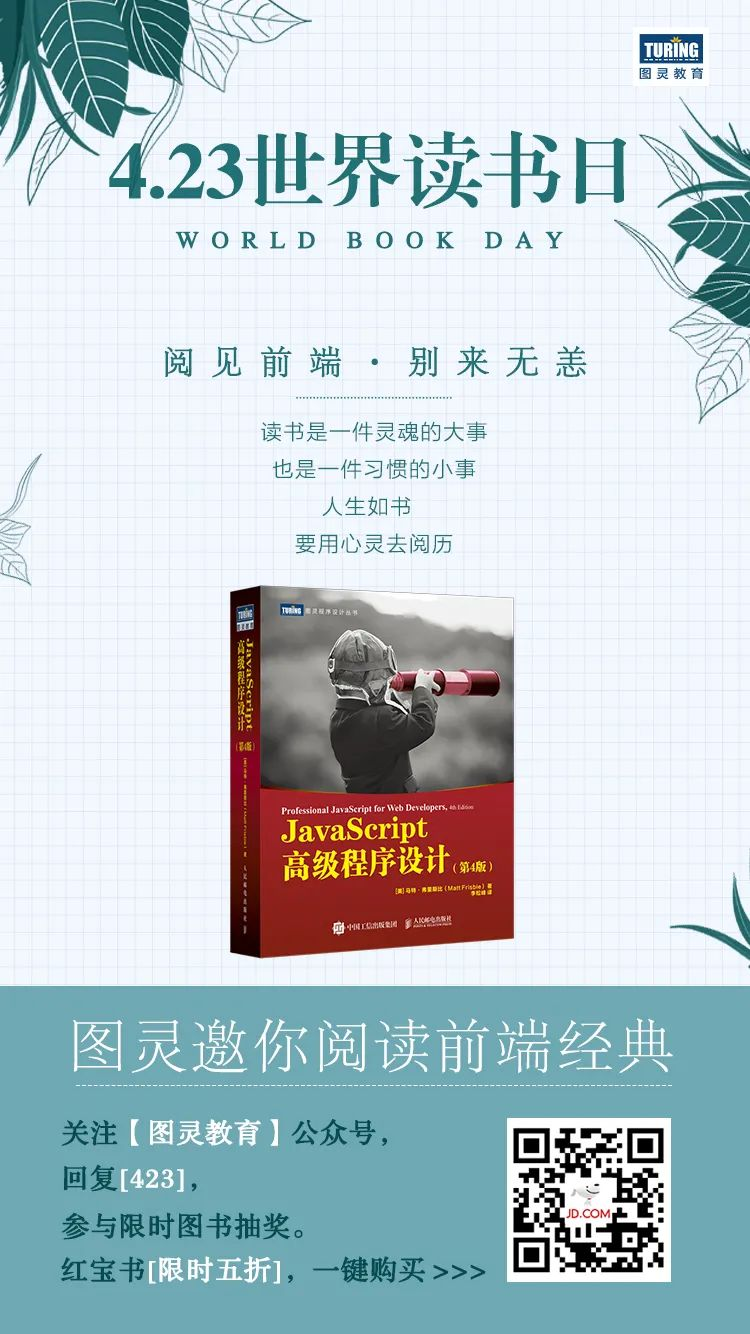## 一文探索 JavaScript 最强大特性—函数表达式

news2023/11/30 8:14:10

``````function sum (num1, num2) {
return num1 + num2;
}
``````

``````let sum = function(num1, num2) {
return num1 + num2;
};
``````

``````let sum = (num1, num2) => {
return num1 + num2;
};
``````

``````let sum = new Function("num1", "num2", "return num1 + num2");  // 不推荐
``````ECMAScript 6 新增了使用胖箭头（=>）语法定义函数表达式的能力。很大程度上，箭头函数实例化的函数对象与正式的函数表达式创建的函数对象行为是相同的。任何可以使用函数表达式的地方，都可以使用箭头函数：

``````let arrowSum = (a, b) => {
return a + b;
};

let functionExpressionSum = function(a, b) {
return a + b;
};

console.log(arrowSum(5, 8)); // 13
console.log(functionExpressionSum(5, 8)); // 13
``````

``````let ints = [1, 2, 3];

console.log(ints.map(function(i) { return i + 1; }));  // [2, 3, 4]
console.log(ints.map((i) => { return i + 1 }));        // [2, 3, 4]
``````

``````// 以下两种写法都有效
let double = (x) => { return 2 * x; };
let triple = x => { return 3 * x; };

// 没有参数需要括号
let getRandom = () => { return Math.random(); };

// 多个参数需要括号
let sum = (a, b) => { return a + b; };

// 无效的写法：
let multiply = a, b => { return a * b; };
``````

``````// 以下两种写法都有效，而且返回相应的值
let double = (x) => { return 2 * x; };
let triple = (x) => 3 * x;

// 可以赋值
let value = {};
let setName = (x) => x.name = "Matt";
setName(value);
console.log(value.name); // "Matt"

// 无效的写法：
let multiply = (a, b) => return a * b;
````````````function sum(num1, num2) {
return num1 + num2;
}

console.log(sum(10, 10));         // 20

let anotherSum = sum;
console.log(anotherSum(10, 10));  // 20

sum = null;
console.log(anotherSum(10, 10));  // 20
``````

ECMAScript 6 的所有函数对象都会暴露一个只读的 name 属性，其中包含关于函数的信息。多数情况下，这个属性中保存的就是一个函数标识符，或者说是一个字符串化的变量名。即使函数没有名称，也会如实显示成空字符串。如果它是使用 Function 构造函数创建的，则会标识成"anonymous"：

``````function foo() {}
let bar = function() {};
let baz = () => {};

console.log(foo.name);               // foo
console.log(bar.name);               // bar
console.log(baz.name);               // baz
console.log((() => {}).name);        //（空字符串）
console.log((new Function()).name);  // anonymous
``````

``````function foo() {}

console.log(foo.bind(null).name);    // bound foo

let dog = {
years: 1,
get age() {
return this.years;
},
set age(newAge) {
this.years = newAge;
}
}

let propertyDescriptor = Object.getOwnPropertyDescriptor(dog, 'age');
console.log(propertyDescriptor.get.name);  // get age
console.log(propertyDescriptor.set.name);  // set age``````ECMAScript 函数的参数跟大多数其他语言不同。ECMAScript 函数既不关心传入的参数个数，也不关心这些参数的数据类型。定义函数时要接收两个参数，并不意味着调用时就传两个参数。你可以传一个、三个，甚至一个也不传，解释器都不会报错。

arguments 对象是一个类数组对象（但不是 Array 的实例），因此可以使用中括号语法访问其中的元素（第一个参数是arguments，第二个参数是arguments）。而要确定传进来多少个参数，可以访问arguments.length属性。

``````function sayHi(name, message) {
console.log("Hello " + name + ", " + message);
}
``````

``````function sayHi() {
console.log("Hello " + arguments + ", " + arguments);
}
``````

``````function howManyArgs() {
console.log(arguments.length);
}

howManyArgs("string", 45);  // 2
howManyArgs();              // 0
howManyArgs(12);            // 1
``````

``````function doAdd() {
if (arguments.length === 1) {
console.log(arguments + 10);
} else if (arguments.length === 2) {
console.log(arguments + arguments);
}
}

``````

``````function doAdd(num1, num2) {
if (arguments.length === 1) {
console.log(num1 + 10);
} else if (arguments.length === 2) {
console.log(arguments + num2);
}
}
``````

arguments 对象的另一个有意思的地方就是，它的值始终会与对应的命名参数同步。来看下面的例子：

``````function doAdd(num1, num2) {
arguments = 10;
console.log(arguments + num2);
}
``````

``````function foo() {
console.log(arguments);
}
foo(5); // 5

let bar = () => {
console.log(arguments);
};
bar(5);  // ReferenceError: arguments is not defined
``````

``````function foo() {
let bar = () => {
console.log(arguments); // 5
};
bar();
}

foo(5);
``````ECMAScript 函数不能像传统编程那样重载。在其他语言比如 Java 中，一个函数可以有两个定义，只要签名（接收参数的类型和数量）不同就行。如前所述，ECMAScript 函数没有签名，因为参数是由包含零个或多个值的数组表示的。没有函数签名，自然也就没有重载。

``````function addSomeNumber(num) {
return num + 100;
}

return num + 200;
}

``````

``````let addSomeNumber = function(num) {
return num + 100;
};

return num + 200;
};

````````````function makeKing(name) {
name = (typeof name !== 'undefined') ? name : 'Henry';
return `King \${name} VIII`;
}

console.log(makeKing());         // 'King Henry VIII'
console.log(makeKing('Louis'));  // 'King Louis VIII'
``````

ECMAScript 6 之后就不用这么麻烦了，因为它支持显式定义默认参数了。下面就是与前面代码等价的ES6写法，只要在函数定义中的参数后面用=就可以为参数赋一个默认值：

``````function makeKing(name = 'Henry') {
return `King \${name} VIII`;
}

console.log(makeKing('Louis'));  // 'King Louis VIII'
console.log(makeKing());         // 'King Henry VIII'
``````

``````function makeKing(name = 'Henry', numerals = 'VIII') {
return `King \${name} \${numerals}`;
}

console.log(makeKing());                 // 'King Henry VIII'
console.log(makeKing('Louis'));          // 'King Louis VIII'
console.log(makeKing(undefined, 'VI'));  // 'King Henry VI'
``````

``````function makeKing(name = 'Henry') {
name = 'Louis';
return `King \${arguments}`;
}

console.log(makeKing());         // 'King undefined'
console.log(makeKing('Louis'));  // 'King Louis'
``````

``````let romanNumerals = ['I', 'II', 'III', 'IV', 'V', 'VI'];
let ordinality = 0;

function getNumerals() {
// 每次调用后递增
return romanNumerals[ordinality++];
}

function makeKing(name = 'Henry', numerals = getNumerals()) {
return `King \${name} \${numerals}`;
}

console.log(makeKing());                // 'King Henry I'
console.log(makeKing('Louis', 'XVI'));  // 'King Louis XVI'
console.log(makeKing());                // 'King Henry II'
console.log(makeKing());                // 'King Henry III'
``````

``````let makeKing = (name = 'Henry') => `King \${name}`;

console.log(makeKing()); // King Henry
``````

``````function makeKing(name = 'Henry', numerals = 'VIII') {
return `King \${name} \${numerals}`;
}

console.log(makeKing()); // King Henry VIII
``````

``````function makeKing() {
let name = 'Henry';
let numerals = 'VIII';

return `King \${name} \${numerals}`;
}
``````

``````function makeKing(name = 'Henry', numerals = name) {
return `King \${name} \${numerals}`;
}

console.log(makeKing()); // King Henry Henry
``````

``````// 调用时不传第一个参数会报错
function makeKing(name = numerals, numerals = 'VIII') {
return `King \${name} \${numerals}`;
}
``````

``````// 调用时不传第二个参数会报错
function makeKing(name = 'Henry', numerals = defaultNumeral) {
let defaultNumeral = 'VIII';
return `King \${name} \${numerals}`;
}``````ECMAScript 6 新增了扩展操作符，使用它可以非常简洁地操作和组合集合数据。扩展操作符最有用的场景就是函数定义中的参数列表，在这里它可以充分利用这门语言的弱类型及参数长度可变的特点。扩展操作符既可以用于调用函数时传参，也可以用于定义函数参数。``````let values = [1, 2, 3, 4];

function getSum() {
let sum = 0;
for (let i = 0; i < arguments.length; ++i) {
sum += arguments[i];
}
return sum;
}
``````

``````console.log(getSum.apply(null, values)); // 10
``````

``````console.log(getSum(...values)); // 10
``````

``````console.log(getSum(-1, ...values));          // 9
console.log(getSum(...values, 5));           // 15
console.log(getSum(-1, ...values, 5));       // 14
console.log(getSum(...values, ...[5,6,7]));  // 28
``````

``````let values = [1,2,3,4]

function countArguments() {
console.log(arguments.length);
}

countArguments(-1, ...values);          // 5
countArguments(...values, 5);           // 5
countArguments(-1, ...values, 5);       // 6
countArguments(...values, ...[5,6,7]);  // 7
``````

arguments 对象只是消费扩展操作符的一种方式。在普通函数和箭头函数中，也可以将扩展操作符用于命名参数，当然同时也可以使用默认参数：

``````function getProduct(a, b, c = 1) {
return a * b * c;
}

let getSum = (a, b, c = 0) => {
return a + b + c;
}

console.log(getProduct(...[1,2]));      // 2
console.log(getProduct(...[1,2,3]));    // 6
console.log(getProduct(...[1,2,3,4]));  // 6

console.log(getSum(...[0,1]));          // 1
console.log(getSum(...[0,1,2]));        // 3
console.log(getSum(...[0,1,2,3]));      // 3
``````

``````function getSum(...values) {
// 顺序累加values中的所有值
// 初始值的总和为0
return values.reduce((x, y) => x + y, 0);
}

console.log(getSum(1,2,3)); // 6
``````

``````// 不可以
function getProduct(...values, lastValue) {}

// 可以
function ignoreFirst(firstValue, ...values) {
console.log(values);
}

ignoreFirst();       // []
ignoreFirst(1);      // []
ignoreFirst(1,2);    // 
ignoreFirst(1,2,3);  // [2, 3]
``````

``````let getSum = (...values) => {
return values.reduce((x, y) => x + y, 0);
}

console.log(getSum(1,2,3)); // 6
``````

``````function getSum(...values) {
console.log(arguments.length);  // 3
console.log(arguments);         // [1, 2, 3]
console.log(values);            // [1, 2, 3]
}

console.log(getSum(1,2,3));````````````// 没问题
console.log(sum(10, 10));
function sum(num1, num2) {
return num1 + num2;
}
``````

``````// 会出错
console.log(sum(10, 10));
let sum = function(num1, num2) {
return num1 + num2;
};
``````

``````console.log(sum(10, 10));
var sum = function(num1, num2) {
return num1 + num2;
};
````````````function callSomeFunction(someFunction, someArgument) {
return someFunction(someArgument);
}
``````

``````function add10(num) {
return num + 10;
}

console.log(result1);  // 20

function getGreeting(name) {
return "Hello, " + name;
}

let result2 = callSomeFunction(getGreeting, "Nicholas");
console.log(result2);  // "Hello, Nicholas"
``````

callSomeFunction() 函数是通用的，第一个参数传入的是什么函数都可以，而且它始终返回调用作为第一个参数传入的函数的结果。要注意的是，如果是访问函数而不是调用函数，那就必须不带括号，所以传给 callSomeFunction() 的必须是 add10 和 getGreeting，而不能是它们的执行结果。

``````function createComparisonFunction(propertyName) {
return function(object1, object2) {
let value1 = object1[propertyName];
let value2 = object2[propertyName];

if (value1 < value2) {
return -1;
} else if (value1 > value2) {
return 1;
} else {
return 0;
}
};
}
``````

``````let data = [
{name: "Zachary", age: 28},
{name: "Nicholas", age: 29}
];

data.sort(createComparisonFunction("name"));
console.log(data.name);  // Nicholas

data.sort(createComparisonFunction("age"));
console.log(data.name);  // Zachary
``````☟☟☟ 红宝书全年最低，只要64.5

### SCAN Learning to Classify Images without Labels(翻译)

SCAN Learning to Classify Images without Labels 概览 Approach: A two-step approach where feature learning and clustering are decoupled. Step 1: Solve a pretext task Mine k nearest neighbrs 通过利用特征相似性来挖掘每张图片的最近邻居nearest neighbors&#…

### MyEclipse图表工具Birt的使用技巧（三）--连接webservice数据源

Web Services 技术是一套标准。它定义了应用程序怎样在Web上实现互操作。用户能够使用不论什么语言。在不同的平台下编写Web Services。然后通过Web Services 的标准来对这些服务进行注冊、查询和訪问。BIRT能够方便的帮助用户訪问基于SOAP的Web Services&#xff0c;而且使用S…

### 安装Ubuntu13.10后必做的10件事

Ubuntu 13.10发布了&#xff0c;而且你已经升级了&#xff0c;然后你想知道现在要做些什么。不要着急&#xff0c;这里有10件安装完Ubuntu 13.10后必做的事。 我们以前为ubuntu每个版本整理了一个安装后核对表&#xff0c;但是因为新功能的到来以及不断的进步&#xff0c;我们建…

### 演讲实录 | DevOps 2021年度洞察

2021DevOpsDays上海站闪耀落幕4月17日的DevOpsDays峰会上海站圆满落幕&#xff0c;众多业内大咖及风云人物悉数亮相&#xff0c;为大家分享了DevOps、精益、敏捷的最新落地实践及案例。全量PPT下载&#xff0c;提取码&#xff1a;3fshhttps://pan.baidu.com/share/init?surlVb…